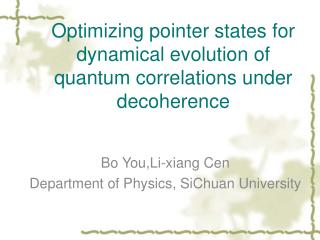# Optimizing pointer states for dynamical evolution of quantum correlations under decoherence - PowerPoint PPT PresentationDownload PresentationOptimizing pointer states for dynamical evolution of quantum correlations under decoherence

Download Presentation## Optimizing pointer states for dynamical evolution of quantum correlations under decoherence

- - - - - - - - - - - - - - - - - - - - - - - - - - - E N D - - - - - - - - - - - - - - - - - - - - - - - - - - -
##### Presentation Transcript

1. Optimizing pointer states for dynamical evolution of quantum correlations under decoherence Bo You,Li-xiang Cen Department of Physics, SiChuan University

2. Outline 1.Dissipative channels and pointer bases 2. Two different optimizations in relation to quantum discord 2.1.Bell-diagonal state 2.2.two-qubit state of two ranks 3. Optimizing pointer bases to achieve maximal condition entropy

3. Dissipative channels and pointer bases Dynamical evolution under dissipative channel In this case of dephasing channel Pointer bases: selected by the system-environment coupling

4. H. Ollivier and W. H. Zurek, PhysRevLett.88.017901 Quantum discord: Where Geometry measure of Quantum Discord: Where is the set of zero-discord. Entanglement of formation: where B.Dakic,V.Vedral, and C. Brukner,Phys. Rev. Lett. 105, 190502

5. Schematic illustration: dependence of decoherence dynamics on pointer bases

6. Motivation and main concerns We investigate the dynamics of decorrelation under different choices of the pointer states of system-reservoir couplings. In detail, we consider a two-qubit system,initially prepared in certain states with non-zero quantum correlations, subjected to local dissipative channels responsible for various pointer states. Dynamical evolution of entanglement, quantum discord,and the mutual information sharing between the two qubits, is depicted. We elucidate various optimizations of the pointer states, e.g., minimization and maximization of the conditional entropy, as well as the geometric optimization via minimizing discord,and analyze the properties of the corresponding behavior of decorrelation.

7. Case 1: Two different optimizations in relation to quantum discord It is proved that quantum discord and its geometry measure can be rewritten as: Where is the projector state achieving the minimize. Therefore we could choose the two projector states as our optimal bases,so that we could get some physics about dissipate process with different optimizations. S.L. Luo and S.S. Fu,PhysRevA.82.034302

8. Bell-diagonal states Thus, the dynamic with the two optimizations is the same for Bell-diagonal states. We can express the state as: For Bell-diagonal state, the measurements for minimizing the quantum discord and geometry measure of QD can be easily calculated, which are the same, that is: S.L.Luo,Phys. Rev. A 77, 042303 (2008)

9. Two qubits state of two ranks The state express as Where The minimizing discord can be obtained by purifying the state to a three-qubit pure state: In the state, the minimal conditional entropy of AC-system is equal to the entanglement of BC-system: L.X.Cen, et.al., Phys. Rev. A 83, 054101 (2011)

10. Therefore, the minimizing conditional entropy of AC-system could be obtained by calculating the EoF of BC-system. Thus through the method conducted by Wootters(phys.rev.let.80.2245),the decomposition achieving the EoF can be deduced. So the measurement corresponding to minimizing conditional entropy can be determined by: Thus the measurement is: where is the normalized vector , and

11. The measurement for minimizing the geometry measure of can be obtained through the method conducted by B.Dakic(Phys.Rev.Lett.105.190502),write as: Where is the normalized eigenvector with the largest eigenvalue of ,and the state is expressed as: It is clear that ,generally,the measurement for minimizing condition entropy is not the one minimizing the geometry distance of discord ,unless it satisfying: but the state is not necessarily the Bell-diagonal state.

12. where dashed line is the evolution with minimal geometry measure of discord,green line quantum discord. the figure shows the correlation evolution in dephasing process, which set:

13. Case 2: Optimizing pointer bases to achieve maximal condition entropy Quantum discord: If we select the pointer bases without “min”, otherwise, with “max” to conditional entropy ,i.e. ,how situation will be? Generally, the evolution of mutual information is slower than above choices, however the evolution of quantum discord has not easy relation, that is , “max” choice doesn’t meaning the slower quantum discord evolution.

14. The left figure show the evolution of quantum correlation of two-qubit of two ranks above,the red line is corresponding to “max” selection, we can see QD line of “max”-selection is the top one,while the mutual information line of “max” selection is the bottle one. The right figure show the evolution of the state with: we can see QD line of “max”-selection is the bottle one,while the mutual information line of “max” selection is also the bottle one.

15. We could consider the amplitude damping channel, which in Kraus operator is: • Select the pointer bases above, generally, we can find the evolution of the quantum discord has increasing region in “max” selection in above setting, showing below: